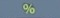# Percents

% is a multiplier.

Basics
% = pct = percent

% = fraction of 100
1% =$\bf\displaystyle\frac{1}{100}$

5% =$\bf\displaystyle\frac{5}{100}$

23% =$\bf\displaystyle\frac{23}{100}$

There is an invisible decimal point between the number and %.
1% = 1.%
5% = 5.%
23% = 23.%

To convert percent to a decimal, move the invisible decimal point two places to the left; this maneuver is the same as dividing the percent by 100.
0.07 ← 7.% = 7%
7 ÷ 100 = 0.07
0.70 ← 70.% = 70%
70 ÷ 100 = 0.70

To convert a decimal to percent, move the decimal point two places to the right;
this maneuver is the same as multiplying the decimal by 100.
0.07 → 7.% = 7%
0.07 × 100 = 7%
0.70 → 70.% = 70%
0.70 × 100 = 70%

% is a multiplier.
The word of is a multiplier.
3% of 500 = 3% × 500is the % command.activatesabove.

Question
Convert 6% to a decimal.

0.06

To convert percent to a decimal, move the invisible decimal point two places to the left.
0.06 ← 6.% = 6%

Question
Convert 65% to a decimal.

0.65

To convert percent to a decimal, move the invisible decimal point two places to the left.
0.65 ← 65.% = 65%

Question
Convert 0.08 to percent.

8%

To convert a decimal to percent, move the decimal point two places to the right.
0.08 → 8.% = 8%

Question
Convert 0.85 to percent.

85%

To convert a decimal to percent, move the decimal point two places to the right.
0.85 → 85.% = 85%

Question
Bob earns 3% interest a year on his savings account.  If he starts the year with $50000 and does not withdraw anything from his savings account, how much interest will Bob earn by the end of the year? Answer$1500

% is a multiplier.
3% × 50000 = 1500

Calculator Click What You See Commentblinker clears screen× 50000 3%*50000 % is a multiplier.1500 Answer
###### Percent

Question
Bob earns 3% interest a year on his savings account.  If he starts the year with $50000 and does not withdraw anything from his savings account, what will Bob’s new balance be at the end of the year? Answer$51500

New Balance = Old Balance + Interest
New Balance = 50000 + 3% × 50000 = 51500

Calculator Click What You See Commentblinker clears screen
50000 + 3× 50000 50000+3%*5000051500 Answer
###### Percent

Question
Bob earns 3% interest a year on his savings account.  The bank imposes a $7.25 fee to withdraw all funds from such an account. If Bob starts the year with$50000 in his savings account and withdraws everything at year-end, how much money will he have?

$3.02 Answer Process 10.00 – 6.50 – 8% × 6.50 + 0.04 = 3.02 Calculator Click What You See Commentblinker clears screen 10.00 – 6.50 –× 6.50 + 0.04 10.00-6.50-8%*6.50+0.043.02 Answer ###### Percent Practice – Questions 1. Convert 9% to a decimal. 2. Convert 95% to a decimal. 3. Convert 0.04 to percent. 4. Convert 0.45 to percent. 5. Convert 0.455 to percent. 6. Betty, a real estate agent, earned a 6% commission on the sale of a$215000 house.
How much did Betty earn?

7.  With reference to Question 1, if, in a particular year, Betty sells only the $215000 house and earns$5000 answering the office phone on the side, how much did she earn that particular year?

8.  A new car costing $27500 depreciates (loses value) by 20% after one year? By how much does the car depreciate after one year? 9. With reference to Question 8, how much is the car worth after one year? 10. Joe’s knee surgery cost$20000.  Because the health insurance company requires a deductible, Joe must pay the first $4000 out-of-pocket. Because the health insurance company also requires a copayment, it will pay only 80% of the remaining balance. How much of the remaining balance will the health insurance company pay? 11. With reference to Question 10, how much of the remaining balance does Joe owe as the copayment? 12. With reference to Questions 10 and 11, how much in total, including both the deductible and copayment, does Joe owe? 13. A rare painting by a famous artist appreciates (increases value) by 15% a year. If the initial value of the painting is$175000, by how much does it appreciate after one year?

14.  With reference to Question 13, how much is the painting worth after one year?

15.  With reference to Questions 13 and 14, how much is the painting worth after two years?

1.  0.09

2.  0.95

3.  4%

4.  45%

5.  45.5%

6.  $12900 7.$17900

8.  $5500 9.$22000

10.  $12800 11.$3200

12.  $7200 13.$26250

14.  $201250 15.$231437.50